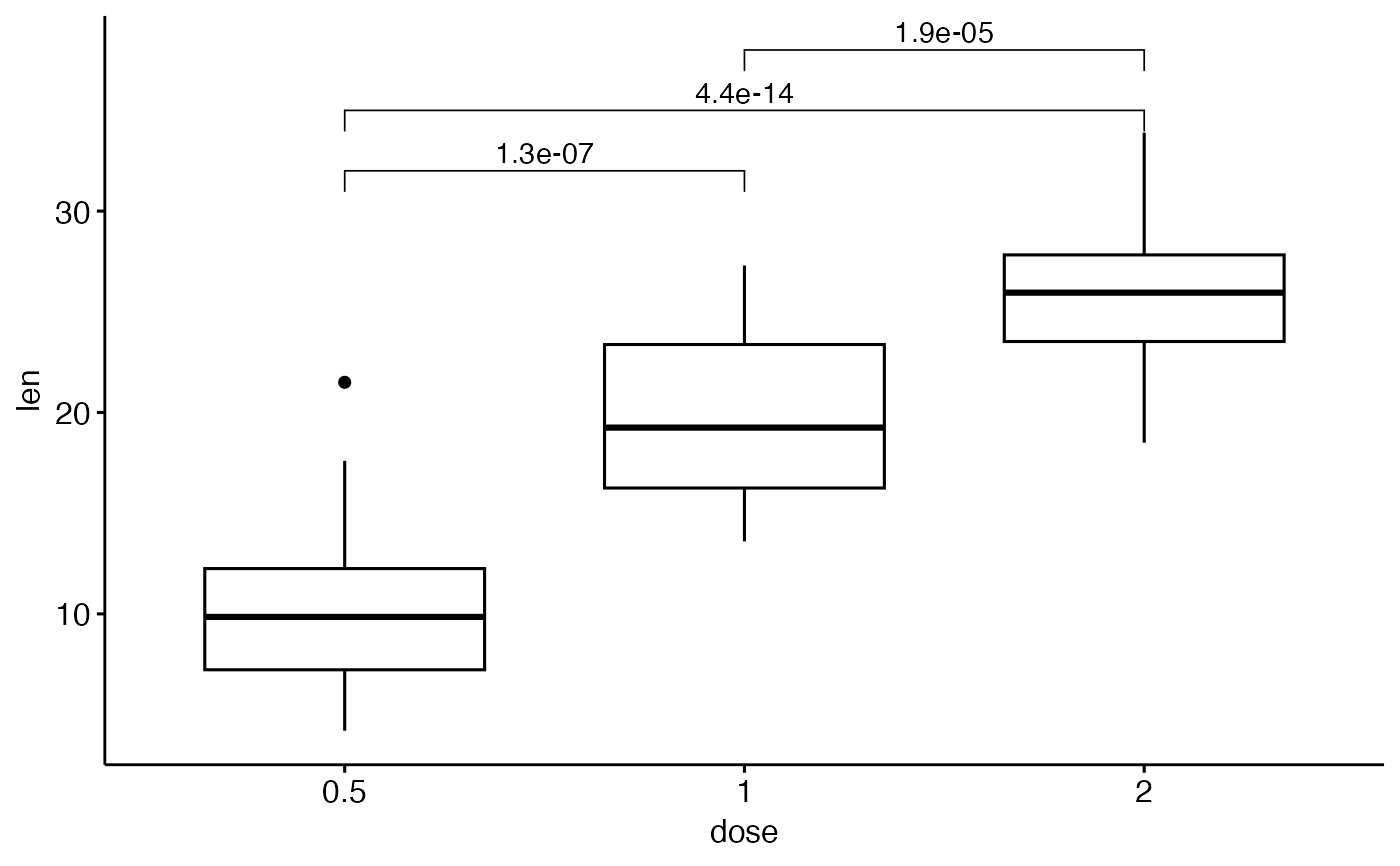add brackets with label annotation to a ggplot. Helpers for adding p-value or significance levels to a plot.

stat_bracket(
mapping = NULL,
data = NULL,
position = "identity",
na.rm = FALSE,
show.legend = NA,
inherit.aes = TRUE,
label = NULL,
type = c("text", "expression"),
y.position = NULL,
xmin = NULL,
xmax = NULL,
step.increase = 0,
step.group.by = NULL,
tip.length = 0.03,
bracket.nudge.y = 0,
bracket.shorten = 0,
size = 0.3,
label.size = 3.88,
family = "",
vjust = 0,
...
)

geom_bracket(
mapping = NULL,
data = NULL,
stat = "bracket",
position = "identity",
na.rm = FALSE,
show.legend = NA,
inherit.aes = TRUE,
label = NULL,
type = c("text", "expression"),
y.position = NULL,
xmin = NULL,
xmax = NULL,
step.increase = 0,
step.group.by = NULL,
tip.length = 0.03,
bracket.nudge.y = 0,
bracket.shorten = 0,
size = 0.3,
label.size = 3.88,
family = "",
vjust = 0,
coord.flip = FALSE,
...
)

## Arguments

mapping

Set of aesthetic mappings created by aes(). If specified and inherit.aes = TRUE (the default), it is combined with the default mapping at the top level of the plot. You must supply mapping if there is no plot mapping.

data

The data to be displayed in this layer. There are three options:

If NULL, the default, the data is inherited from the plot data as specified in the call to ggplot().

A data.frame, or other object, will override the plot data. All objects will be fortified to produce a data frame. See fortify() for which variables will be created.

A function will be called with a single argument, the plot data. The return value must be a data.frame, and will be used as the layer data. A function can be created from a formula (e.g. ~ head(.x, 10)).

position

Position adjustment, either as a string naming the adjustment (e.g. "jitter" to use position_jitter), or the result of a call to a position adjustment function. Use the latter if you need to change the settings of the adjustment.

na.rm

If FALSE (the default), removes missing values with a warning. If TRUE silently removes missing values.

show.legend

logical. Should this layer be included in the legends? NA, the default, includes if any aesthetics are mapped. FALSE never includes, and TRUE always includes. It can also be a named logical vector to finely select the aesthetics to display.

inherit.aes

If FALSE, overrides the default aesthetics, rather than combining with them. This is most useful for helper functions that define both data and aesthetics and shouldn't inherit behaviour from the default plot specification, e.g. borders().

label

character vector with alternative label, if not null test is ignored

type

the label type. Can be one of "text" and "expression" (for parsing plotmath expression).

y.position

numeric vector with the y positions of the brackets

xmin

numeric vector with the positions of the left sides of the brackets

xmax

numeric vector with the positions of the right sides of the brackets

step.increase

numeric vector with the increase in fraction of total height for every additional comparison to minimize overlap.

step.group.by

a variable name for grouping brackets before adding step.increase. Useful to group bracket by facet panel.

tip.length

numeric vector with the fraction of total height that the bar goes down to indicate the precise column

bracket.nudge.y

Vertical adjustment to nudge brackets by. Useful to move up or move down the bracket. If positive value, brackets will be moved up; if negative value, brackets are moved down.

bracket.shorten

a small numeric value in [0-1] for shortening the with of bracket.

size

change the width of the lines of the bracket

label.size

change the size of the label text

family

change the font used for the text

vjust

move the text up or down relative to the bracket

...

other arguments passed on to layer. These are often aesthetics, used to set an aesthetic to a fixed value, like color = "red" or size = 3. They may also be parameters to the paired geom/stat.

stat

The statistical transformation to use on the data for this layer, either as a ggproto Geom subclass or as a string naming the stat stripped of the stat_ prefix (e.g. "count" rather than "stat_count")

coord.flip

logical. If TRUE, flip x and y coordinates so that horizontal becomes vertical, and vertical, horizontal. When adding the p-values to a horizontal ggplot (generated using coord_flip()), you need to specify the option coord.flip = TRUE.

## Examples

df <- ToothGrowth
df$dose <- factor(df$dose)

ggboxplot(df, x = "dose", y = "len") +
geom_bracket(
xmin = "0.5", xmax = "1", y.position = 30,
label = "t-test, p < 0.05"
)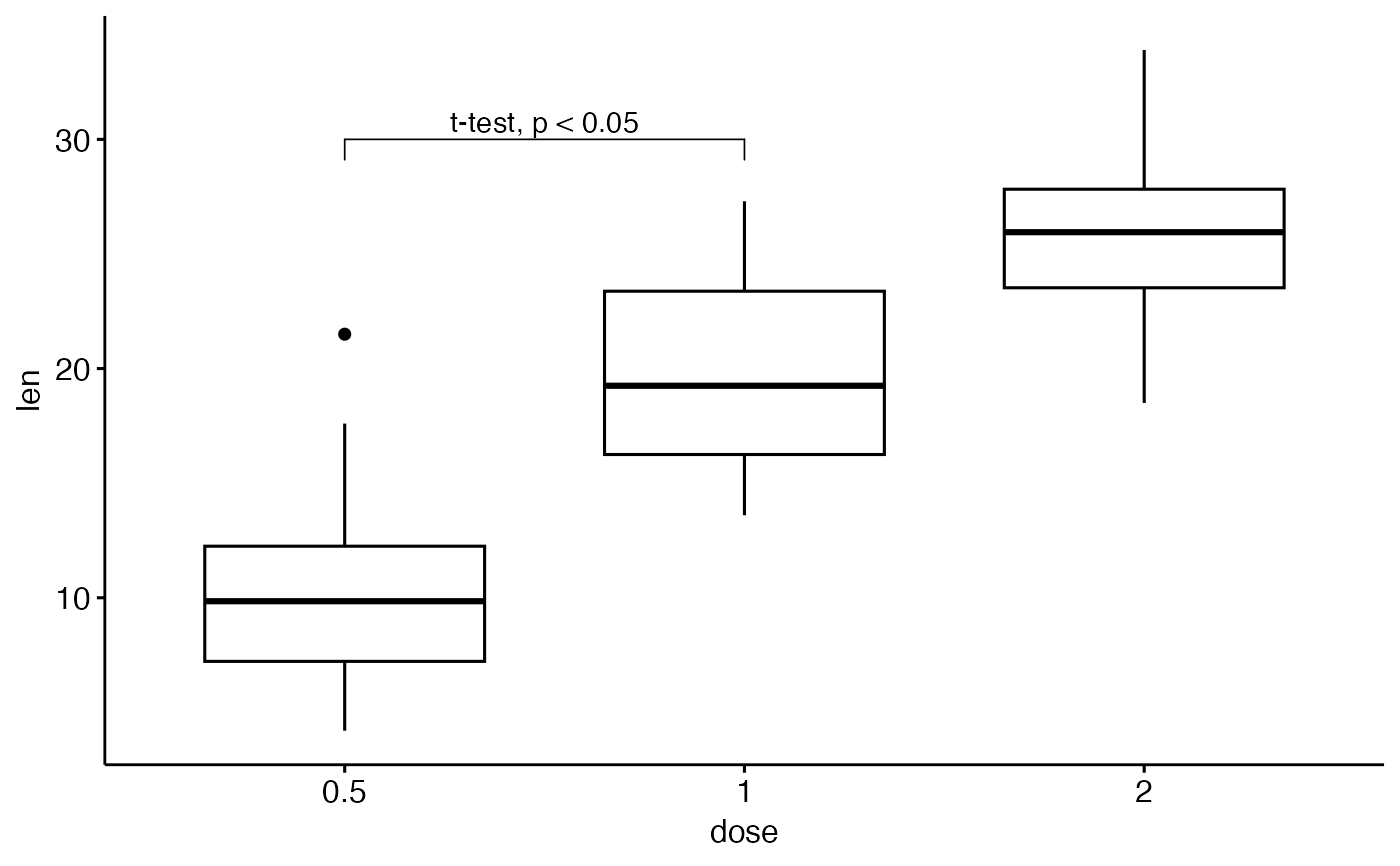# Customize bracket tip.length tip.length
ggboxplot(df, x = "dose", y = "len") +
geom_bracket(
xmin = "0.5", xmax = "1", y.position = 30,
label = "t-test, p < 0.05", tip.length = c(0.2, 0.02)
)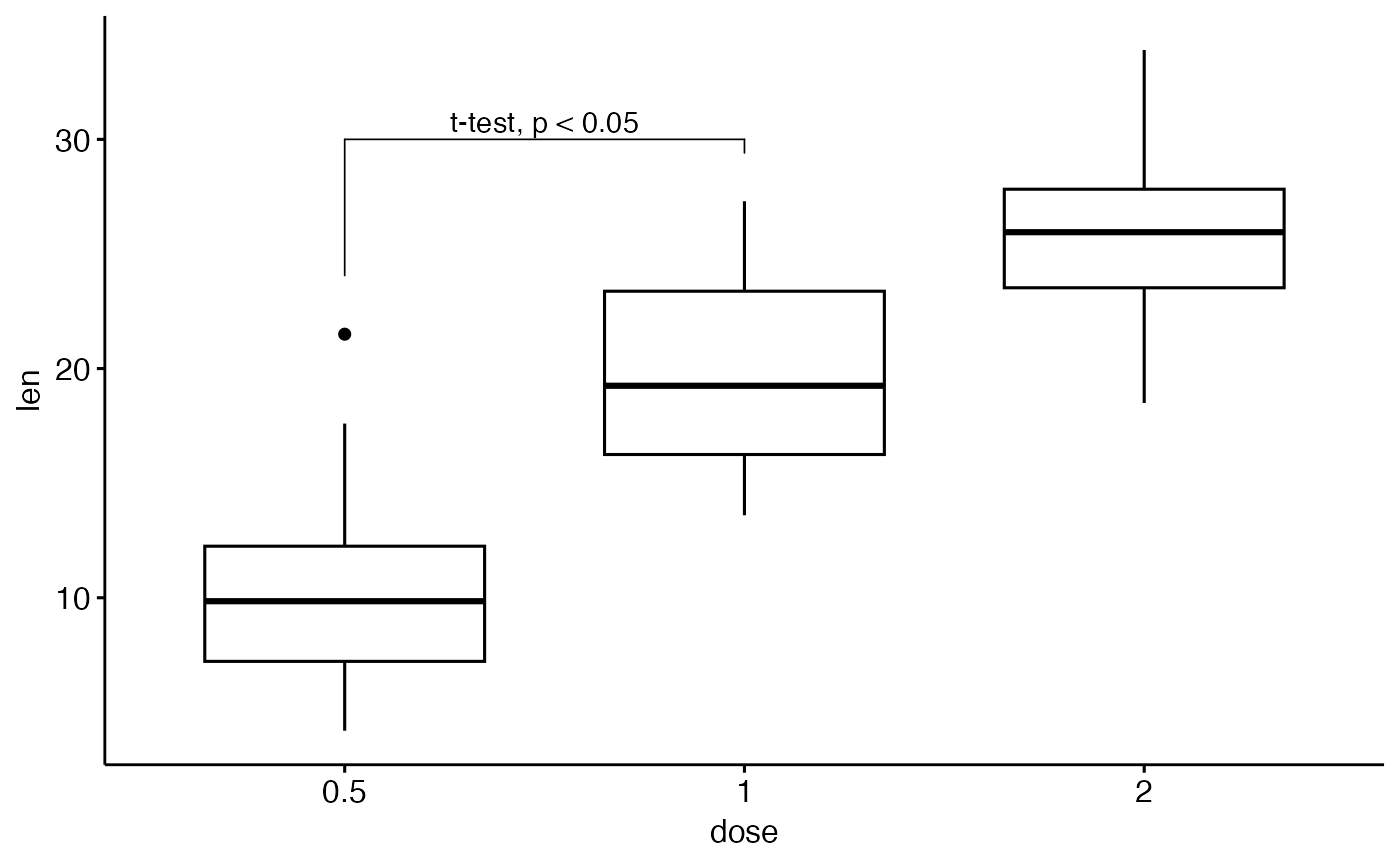#Using plotmath expression
ggboxplot(df, x = "dose", y = "len") +
geom_bracket(
xmin = "0.5", xmax = "1", y.position = 30,
label = "list(~italic(p)<=0.001)", type = "expression",
tip.length = c(0.2, 0.02)
)# Specify multiple brackets manually
ggboxplot(df, x = "dose", y = "len") +
geom_bracket(
xmin = c("0.5", "1"), xmax = c("1", "2"),
y.position = c(30, 35), label = c("***", "**"),
tip.length = 0.01
)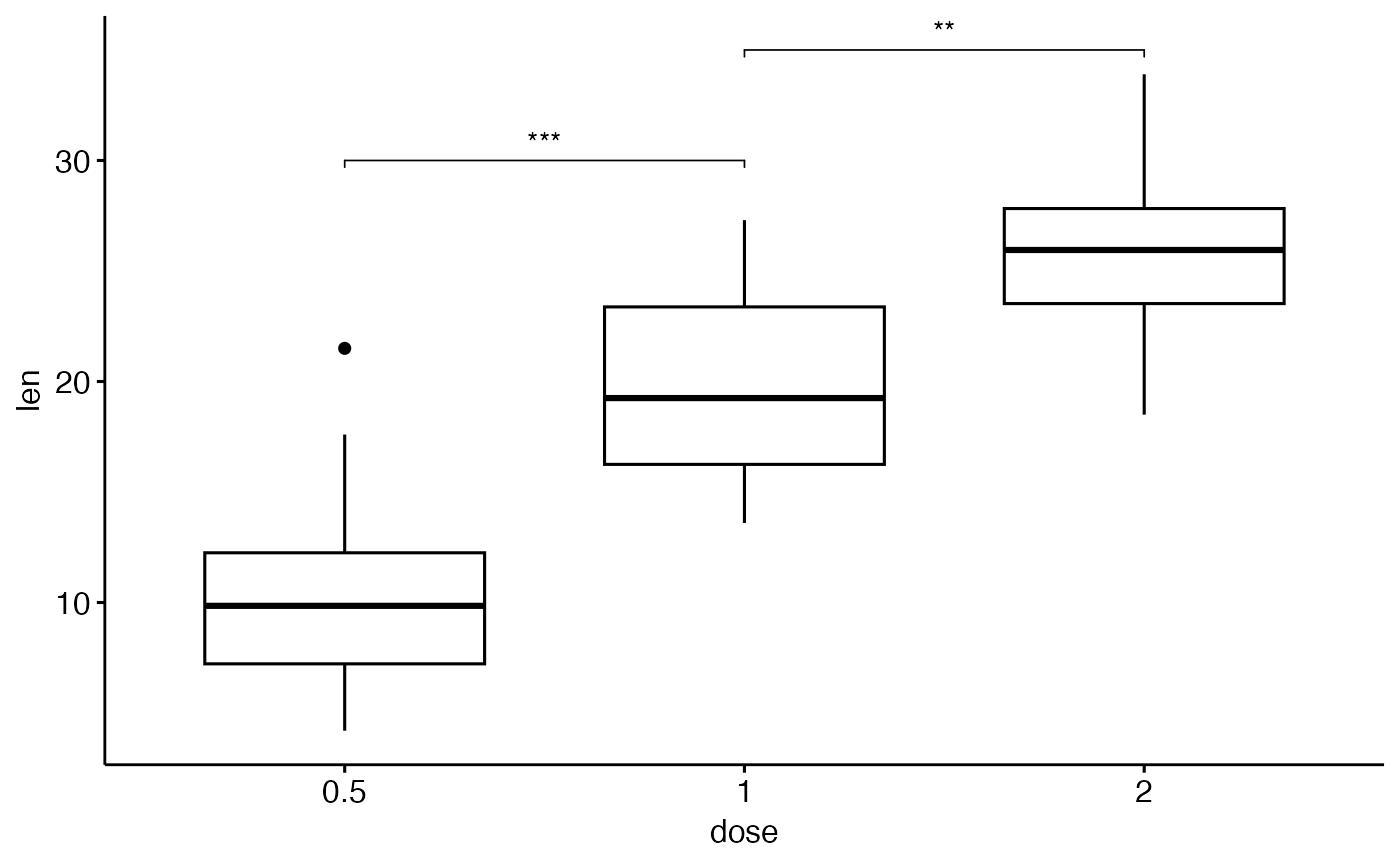# Compute statistical tests and add p-values
stat.test <- compare_means(len ~ dose, ToothGrowth, method = "t.test")
ggboxplot(df, x = "dose", y = "len") +
geom_bracket(
aes(xmin = group1, xmax = group2, label = signif(p, 2)),
data = stat.test, y.position = 35
)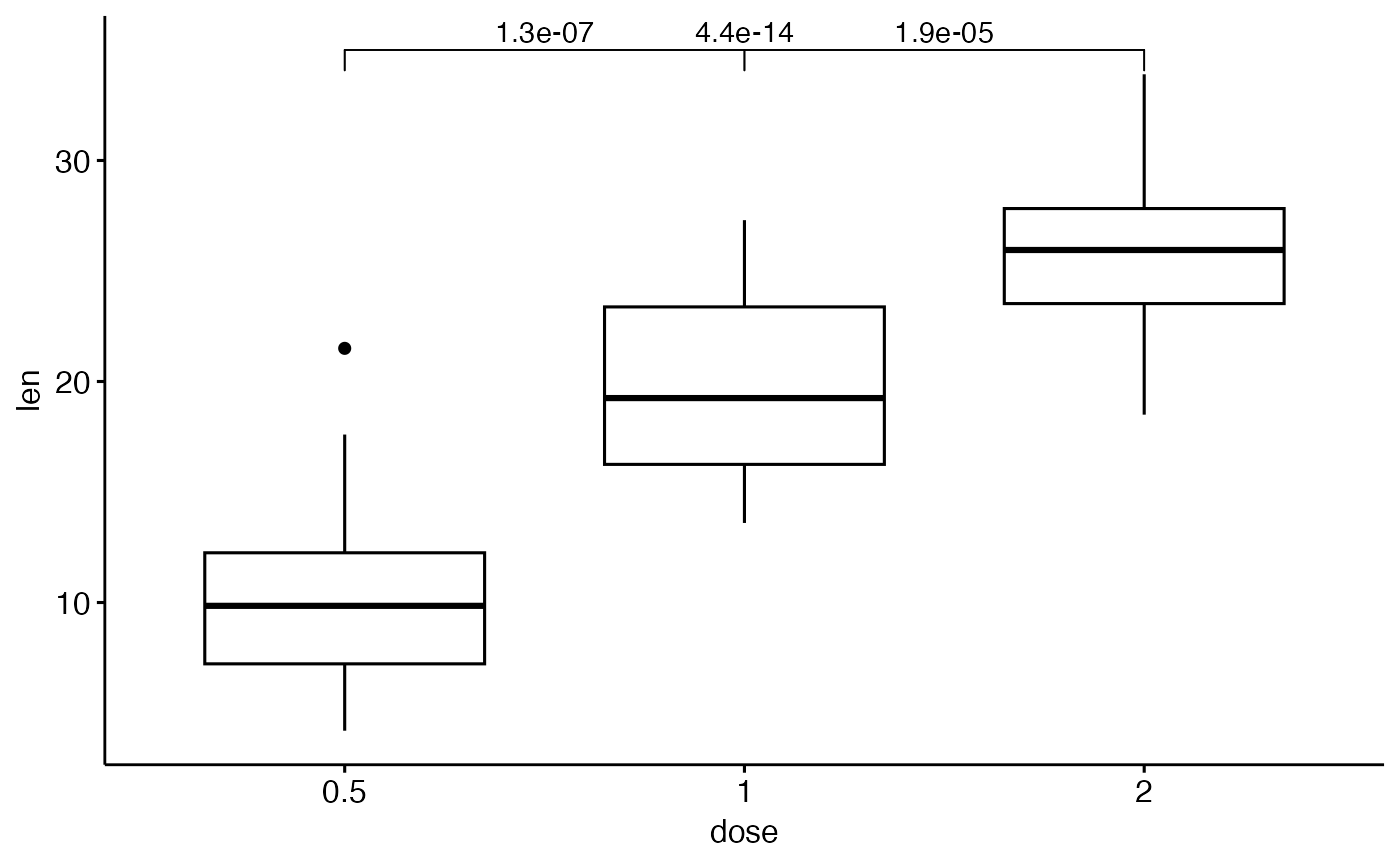# Increase step length between brackets
ggboxplot(df, x = "dose", y = "len") +
geom_bracket(
aes(xmin = group1, xmax = group2, label = signif(p, 2)),
data = stat.test, y.position = 35, step.increase = 0.1
)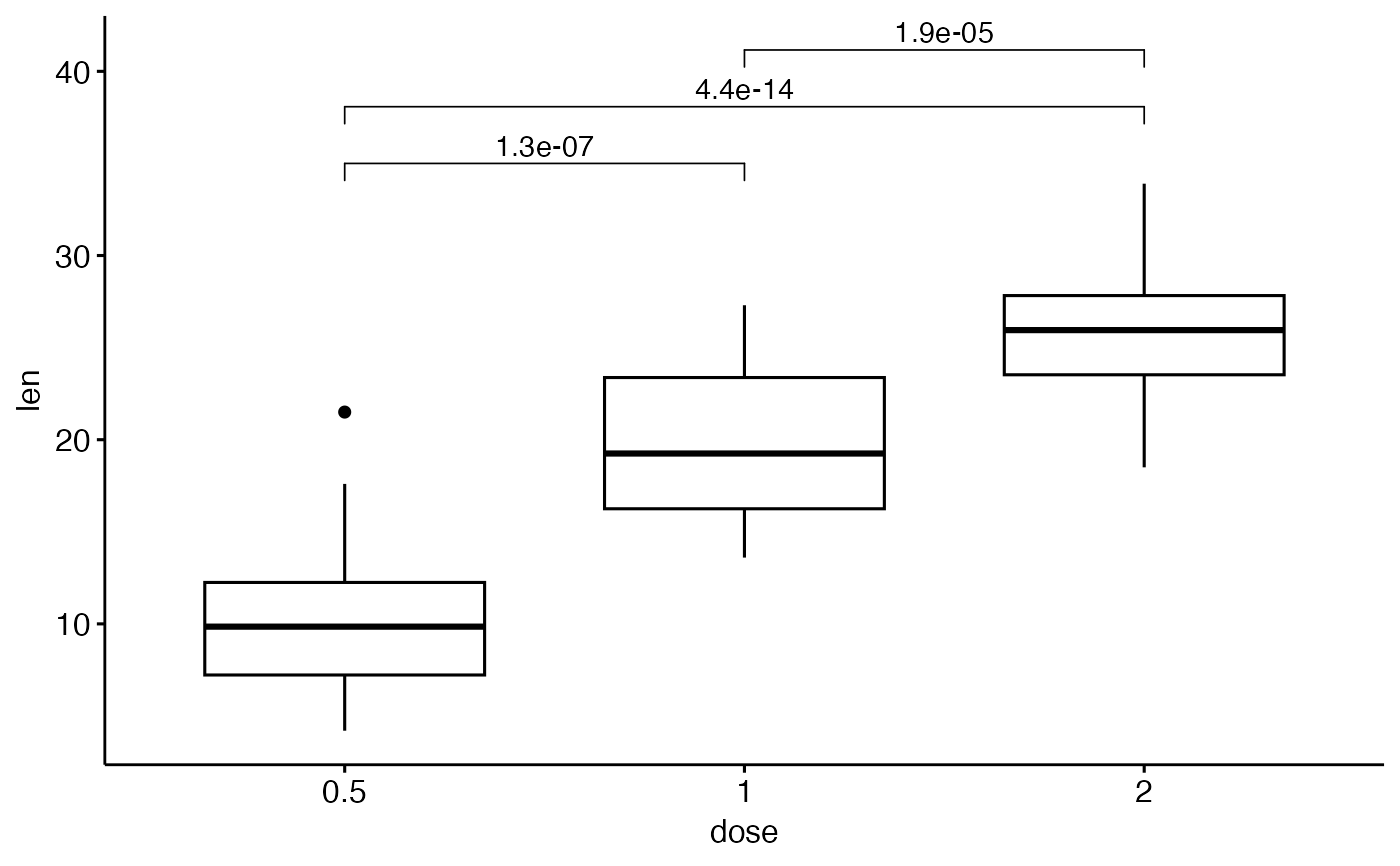# Or specify the positions of each comparison
ggboxplot(df, x = "dose", y = "len") +
geom_bracket(
aes(xmin = group1, xmax = group2, label = signif(p, 2)),
data = stat.test, y.position = c(32, 35, 38)
)